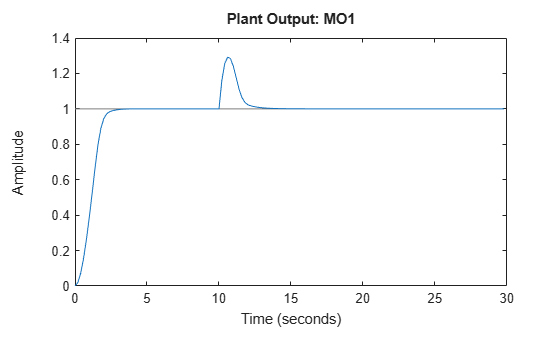# sim

Simulate closed-loop/open-loop response to arbitrary reference and disturbance signals for implicit or explicit MPC

## Syntax

```sim(MPCobj,T,r) sim(MPCobj,T,r,v) sim(___,SimOptions) [y,t,u,xp,xmpc,SimOptions] = sim(___) ```

## Description

Use `sim` to simulate the implicit (traditional) or explicit MPC controller in closed loop with a linear time-invariant model, which, by default, is the plant model contained in `MPCobj.Model.Plant`. As an alternative, `sim` can simulate the open-loop behavior of the model of the plant, or the closed-loop behavior in the presence of a model mismatch, when the controller’s prediction model differs from the actual plant model.

`sim(MPCobj,T,r)` simulates the closed-loop system formed by the plant model specified in `MPCobj.Model.Plant` and by the MPC controller specified by the MPC controller `MPCobj`, in response to the specified reference signal, `r`. The MPC controller can be either a traditional MPC controller (`mpc`) or explicit MPC controller (`explicitMPC`). The simulation runs for the specified number of simulation steps, `T`. `sim` plots the simulation results.

`sim(MPCobj,T,r,v)` also specifies the measured disturbance signal `v`.

`sim(___,SimOptions)` specifies additional simulation options. This syntax allows you to alter the default simulation options, such as initial states, input/output noise and unmeasured disturbances, plant mismatch, etc. You can use `SimOptions` with any of the previous input combinations.

```[y,t,u,xp,xmpc,SimOptions] = sim(___)``` suppresses plotting and instead returns the sequence of plant outputs `y`, the time sequence `t` (equally spaced by `MPCobj.Ts`), the manipulated variables `u` generated by the MPC controller, the sequence `xp` of states of the model of the plant used for simulation, the sequence `xmpc` of states of the MPC controller (provided by the state observer), and the simulation options, `SimOptions`. You can use this syntax with any of the allowed input argument combinations.

## Input Arguments

 `MPCobj` MPC controller containing the parameters of the Model Predictive Control law to simulate, specified as either an implicit MPC controller (`mpc`) or an explicit MPC controller (`explicitMPC`). `T` Number of simulation steps, specified as a positive integer. If you omit `T`, the default value is the row size of whichever of the following arrays has the largest row size: The input argument `r`The input argument `v`The `UnmeasuredDisturbance` property of `SimOptions`, if specifiedThe `OutputNoise` property of `SimOptions`, if specified Default: The largest row size of `r`, `v`, `UnmeasuredDisturbance`, and `OutputNoise` `r` Reference signal, specified as an array. This array has `ny` columns, where `ny` is the number of plant outputs. `r` can have anywhere from 1 to `T` rows. If the number of rows is less than `T`, the missing rows are set equal to the last row. Default: `MPCobj.Model.Nominal.Y` `v` Measured disturbance signal, specified as an array. This array has `nv` columns, where `nv` is the number of measured input disturbances. `v` can have anywhere from 1 to `T` rows. If the number of rows is less than `T`, the missing rows are set equal to the last row. Default: Corresponding entries from `MPCobj.Model.Nominal.U` `SimOptions` Simulation options, specified as an `mpcsimopt` object. Default: `[]`

## Output Arguments

 `y` Sequence of controlled plant outputs, returned as a `T`-by-`Ny` array, where `T` is the number of simulation steps and `Ny` is the number of plant outputs. The values in `y` include neither additive output disturbances nor additive measurement noise (if any). `t` Time sequence, returned as a `T`-by-1 array, where `T` is the number of simulation steps. The values in `t` are equally spaced by `MPCobj.Ts`. `u` Sequence of manipulated variables generated by the MPC controller, returned as a `T`-by-`Nu` array, where `T` is the number of simulation steps and `Nu` is the number of manipulated variables. `xp` Sequence of plant model states, `T`-by-`Nxp` array, where `T` is the number of simulation steps and `Nxp` is the number of states in the plant model. The plant model is either `MPCobj.Model` or `SimOptions.Model`, if the latter is specified. `xmpc` Sequence of MPC controller state estimates, returned as a `T`-by-1 structure array. Each entry in the structure array has the same fields as an `mpcstate` object. The state estimates include plant, disturbance, and noise model states at each time step. `SimOptions` Simulation options used, returned as an `mpcsimopt` object.

## Examples

collapse all

Simulate the MPC control of a MISO system. The system has one manipulated variable, one measured disturbance, one unmeasured disturbance, and one output.

Create the continuous-time plant model. This plant will be used as the prediction model for the MPC controller.

`sys = ss(tf({1,1,1},{[1 .5 1],[1 1],[.7 .5 1]}));`

Discretize the plant model using a sampling time of 0.2 units.

```Ts = 0.2; sysd = c2d(sys,Ts);```

Specify the MPC signal type for the plant input signals.

`sysd = setmpcsignals(sysd,'MV',1,'MD',2,'UD',3);`

Create an MPC controller for the `sysd` plant model. Use default values for the weights and horizons.

`MPCobj = mpc(sysd);`
```-->The "PredictionHorizon" property of "mpc" object is empty. Trying PredictionHorizon = 10. -->The "ControlHorizon" property of the "mpc" object is empty. Assuming 2. -->The "Weights.ManipulatedVariables" property of "mpc" object is empty. Assuming default 0.00000. -->The "Weights.ManipulatedVariablesRate" property of "mpc" object is empty. Assuming default 0.10000. -->The "Weights.OutputVariables" property of "mpc" object is empty. Assuming default 1.00000. ```

Constrain the manipulated variable to the `[0 1]` range.

`MPCobj.MV = struct('Min',0,'Max',1);`

Specify the simulation stop time.

`Tstop = 30;`

Define the reference signal and the measured disturbance signal.

```num_sim_steps = round(Tstop/Ts); r = ones(num_sim_steps,1); v = [zeros(num_sim_steps/3,1); ones(2*num_sim_steps/3,1)];```

The reference signal, `r`, is a unit step. The measured disturbance signal, `v`, is a unit step, with a 10 unit delay.

Simulate the controller.

`sim(MPCobj,num_sim_steps,r,v)`
```-->The "Model.Disturbance" property of "mpc" object is empty: Assuming unmeasured input disturbance #3 is integrated white noise. Assuming no disturbance added to measured output channel #1. -->The "Model.Noise" property of the "mpc" object is empty. Assuming white noise on each measured output channel. ```